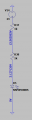# LED circuit, can't explain voltage

#### digitsboy

Joined Dec 29, 2016
38
Hello,
I am a beginner in electronics and i try to understand the circuit below. But i don't understand why the voltage between the resistors is 13.56 volts?
because the difference in voltage between the diode en the powersupply is: 24-3=21 so the voltage in between the resistances must be 10.5 right?
So i don't understand why it is 13.56? Hopefully someone can help me with this! thanks in advance!#### bertus

Joined Apr 5, 2008
20,445
Hello,

The led voltage is about 3.13 Volts. You have used 3 Volts in your calculation.
When you use the 3.13, you will get a total drop of 24-3.13 = 20.87.
Divided by two will give you 10.435.
When you subtract the 10.435 from the 24, you will get 13.565 Volts, close to the value of the simulation.

Bertus

•digitsboy

#### crutschow

Joined Mar 14, 2008
24,709
i don't understand why it is 13.56?
That's the voltage to ground, not the voltage across the resistor.

•bertus

#### Jony130

Joined Feb 17, 2009
5,155

#### digitsboy

Joined Dec 29, 2016
38
ahh stupid haha. ofcourse. Sorry for asking!

One last question. I took a random LED but i saw in the datasheet the forward voltage is inbetween 3.6 and 4V. In the simulation it is 3.1. Is that due to the simulation or something else?

#### Jony130

Joined Feb 17, 2009
5,155
I took a random LED but i saw in the datasheet the forward voltage is inbetween 3.6 and 4V. In the simulation it is 3.1. Is that due to the simulation or something else?
This is normal because 3.6V is just a typical value (just like a average men height) at ID = 20mA and T = 25°C. And the datasheet do not give us any information about the typical Min value.

#### BobTPH

Joined Jun 5, 2013
2,345
ahh stupid haha. ofcourse. Sorry for asking!

One last question. I took a random LED but i saw in the datasheet the forward voltage is inbetween 3.6 and 4V. In the simulation it is 3.1. Is that due to the simulation or something else?
At what current? The Vfw is specified at a specific current. At other currents it will be different.

Bob